# How to use variable in awk command

Variables are used to store any value temporary in any programming language.  Defining the variable in awk command is similar to bash scripting language and it works like bash when the shell variable is used with a single quote and double quote. Awk command has many built-in variables for various purposes. How user-defined, built-in and shell variables can be used in awk command is shown in this tutorial by using different examples.

### Example -1: Defining and printing variable

`awk` command uses ‘-v’ option to define the variable. In this example, the myvar variable is defined in the `awk` command to store the value, “AWK variable” that is printed later. Run the following command from the terminal to check the output.

\$ echo | awk -v myvar='AWK variable' '{print myvar}'

Output: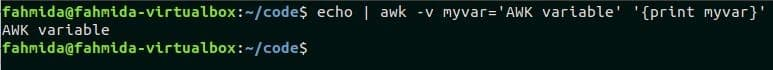### Example – 2: Using shell variable in awk with a single quote and double quote

The example shows how the shell variable can be used `awk` command. Here, a shell variable, myvar is declared with the value, “Linux Hint” in the first command. ‘\$’ symbol is used with a shell variable name to read the value. The second command reads the variable, \$myval with a single quote(‘) and the third command reads the variable \$myvar with double quote(“) in the `awk` statement.

\$ myvar="Linux Hint"
\$ echo | awk -v awkvar='\$myvar' '{ print awkvar; }'
\$ echo | awk -v awkvar="\$myvar" '{ print awkvar; }'

Output:

It is shown in the output that the value of \$myvar can’t be read when it is enclosed with a single quote (‘) and the output is \$myvar. The value of \$myvar is printed when it is enclosed with a double quote (“).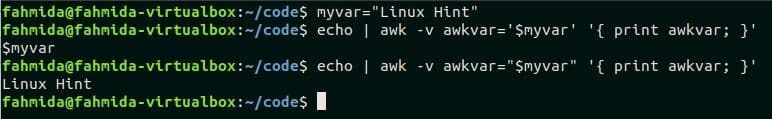### Example – 3: Reading ARGC variable in awk

ARGC variable is used to count the total number of command line arguments. Three command line arguments variables (t1, t2, t3) are passed in the following awk script. Here, the total number of arguments with the script is 4. Run the script from the terminal.

\$ awk 'BEGIN{print "Total arguments=",ARGC}' t1 t2 t3

Output:

The following output will appear after running the script.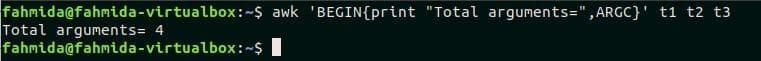### Example – 4: Reading file content by argument variables

Create a text file named customer.txt with the following content to practice this example. Here, every field of the file is separated by single tab space.

customer.txt

ID        Name
103847    John Micheal
209485    Watson
974732    Mira Hossain

Awk command can read each field from any text file by argument variables. There are two fields in customer.txt file. These are ID and Name. The following script will print these two fields by argument variables, \$1 and \$2 by separating two tab spaces. Run the script from the terminal.

\$ cat customer.txt
\$ cat customer.txt | awk '{ print \$1 "\t\t" \$2;}'

Output:

The following output will appear after running the above commands.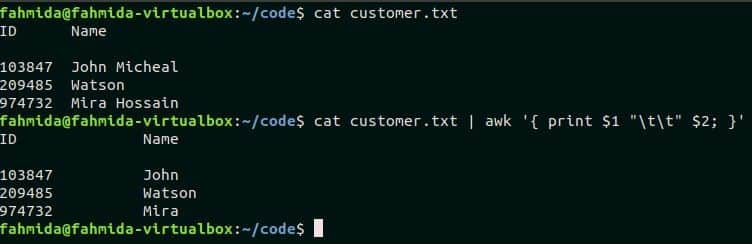### Example- 5: Using built-in variable, FS and field separator option with awk command

FS variable is used in awk command as a field separator. Space is used as a default value of FS. The following command will read the file customer.txt using space as field separator and print the file content. Run the command from the terminal.

\$ awk FS customer.txt

Output:

The following output will appear after running the script.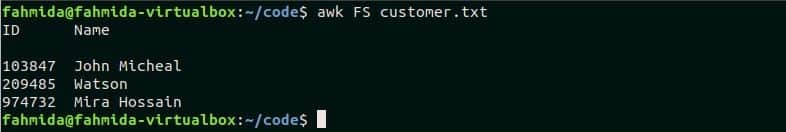Awk command can use other characters as field separator by using the ‘-F’ option. Create a text file named product.txt with the following content where ‘:’ is used as a field separator.

product.txt

101:Cake:\$30
102:Pencil:\$5
103:Soap:\$3
104:Shampoo:\$10

There are three fields in the file, product.txt that contains product id, name and price. The following awk command will print only the second field of each line. Run the commands from the terminal.

\$ cat product.txt
\$ awk -F ':' '{ print \$2 }' product.txt

Output:

Here, the first command printed the content of product.txt and the second command printed only the second field of the file.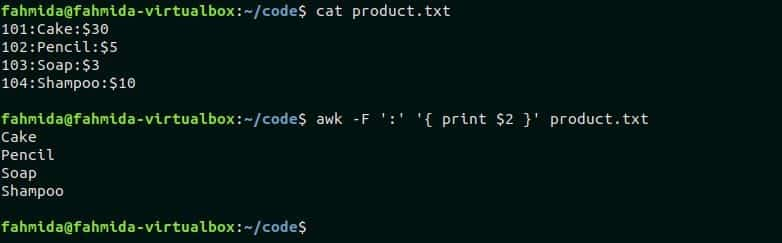### Example – 6: Using built-in variable, NR with awk command

NR variable is used in awk command to count the total number of records or lines of a file. Create a text file named student.txt to test the function of this variable.

student.txt

Name      Batch     Semester
John      20        3
Mira      22        1
Ella      18
Charle    15        8

The following awk script will print the first three lines of product.txt file. Here, a condition is added by using the NR variable. The command will print those lines where the NR value is less than 4. Run the script from the terminal.

Output:

The following output will appear after running the script.

\$ cat student.txt | awk 'NR < 4'### Example – 7: Using built-in variable, NF with awk command

NF variable is used in awk command to count the total number of fields in each line of a file. The following awk script is applied for the file, student.txt which is created in the previous example. The script will print those lines from student.txt file where the total fields are less than 3. Run the command from the terminal.

\$ cat student.txt | awk 'NF < 3'

Output:

There is just one line exists in the file where the total number of fields is less than 3 that is printed as output.### Example – 8: Using built-in variable, OFS with awk command

OFS variable is used in awk command to add output field separator in the output. product.txt file is used in this example to show the use of OFS variable. ‘:’ is used as field separator in product.txt file. The following awk script used ‘->’ as OFS value and, the second and the third fields of the file will print by adding this separator. Run the commands from the terminal.

\$ cat product.txt
\$ awk -F ':' 'BEGIN{OFS="->";} {print \$2,\$3;}' product.txt

Output:

The following output will print after running the commands.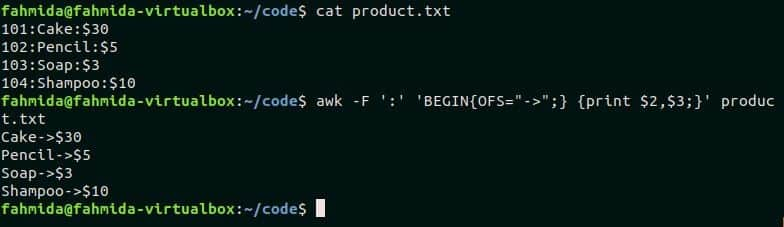#### Conclusion:

Most common uses of awk variables are tried to explain in this tutorial. Hope, the reader will be able to use awk variables properly in the script after practicing this tutorial.